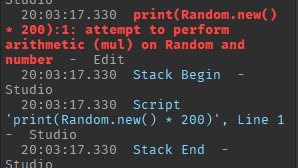# What is better for chances?

Hello. I’m using this as the following for a chance:

``````			local secretChance = math.random(1, 1000000000)
if secretChance == 1 then
winner = 10760425;
game.ReplicatedStorage.Chat:FireAllClients(player.Name .. ' has unboxed a secret item! The Staff of Sparks! (0.000000001' .. '%)','SourceSansBold')
end
``````

Is there a more accurate way to get the chances I want?

I can’t think of a more accurate way. But, there’s probably one out there.

I wanted to try Random.new() but I’m not sure how to use Random and if it’s more accurate.

You could use `Random.new():NextNumber(1, 1000000000)` though I haven’t used it myself so I’m not sure if this will work, but you can give it a try and let me know.

Chance weights are another way of getting chances of items

If you wanted a RREEALLLY precise number, you could use this:

``````local number = 200
print(Random.new() * number)
--Random.new creates a random number inbetween 1 and 0 as a float.
--Integer is what it is being multiplied by, as our limit.
``````

That seems like an overcomplicated function for what `math.random()` can do.

It’s more precise I believe but personally, I would stick to `math.random()` for simplicity.

You could also do `Random.new() * number` as well.Oh wait. I was going off of the javascript random. From what I researched, it’s actually `Random:NextNumber() * 200`

Wait. that still doesn’t work. Dang, I think math.random really is the best way.

try Random.new():NextNumber() * 200

Ok, I fixed it now. It’s actually:

``````Random.new():NextNumber() * number
``````

This results in the following code:

``````local number = 200
print(Random.new():NextNumber() * number)
--Random.new creates a random number inbetween 1 and 0 as a float.
--Integer is what it is being multiplied by, as our limit.
--Example output: 195.1827561742793...
``````